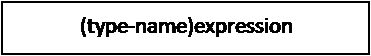My Blog
Articles related to programming, computer science, technology and research.
22/04/2016 Categories: C Programming. Comments Off on Type Conversion and Casting

Converting a data type of an expression to another data type is known as type conversion. Here expression refers to either a variable or value or sub expression. There are two types of conversions. They are:

1. Implicit conversion or Coercion
2. Explicit conversion or Casting

## Implicit Conversion

While evaluating expressions, it is common to have operands of different data types. C automatically converts the data type of operands or any intermediate values to the proper type so that expression can be evaluated without losing any significance. This automatic conversion performed by C is known as implicit conversion.

In C, the expression on the right hand side of an assignment statement is evaluated first. So, C evaluates the expression based on the conversion hierarchy as shown in the below diagram. Finally, the type of the result acquired from the expression is again converted to match the type of variable on the left hand side. The conversion hierarchy is as shown below:### Example

Let’s try to evaluate the following expression:

x = l / i + i * f – d

where, i and x are of the type int, f is of type float, d is of type double and l is of type long int. When the above expression is evaluated, the operand i in the sub expression l/i, is converted to long int since, l is a long int. In the sub expression i*f, i is converted into float, since f is of the type float. The result of the sub expression l/i is converted to float, as it will be added to the result of i*f which is already a float. Finally the result of the sub expression l/i+i*f is converted into double, as d is a double. After obtaining the final result which is of the type double, it is again converted into int as the variable x is of the type int.

## Explicit Conversion or Casting

In C programming, there will be instances where a programmer wants to change the type of an expression into another type which is not possible by the implicit conversion. In such cases, the programmer himself/herself specifies the type conversion. Such conversion is known as explicit conversion or type casting.

For example, let num1 and num2 are variables of integer type and value of num1 is 3 and value of num2 is 2. For the purpose of calculating the average of num1 and num2, let us declare another variable result of the type float. So the statement for calculating the average will be as shown below:

result =( num1+num2)/2

Mathematically the result of the above expression should be 2.5. But when we execute the above statement in a C program, we get the result 2.000000. Even though C applies implicit conversion from int to float, the result we obtained is wrong. In such case we apply explicit casting. The statement will be as follows:

result = (float)(num1+num2)/2

Now, the result of num1+num2 is converted into float. Since one operand is of type float, 2 will also be converted to float. The result of the whole expression on the right hand side is a float. Now, we obtain the correct value in the variable result which will be 2.500000.

The general syntax for explicit type casting is as shown below:Scroll Up